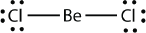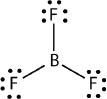# 7.6: Violations of the Octet Rule

•• Anonymous
• LibreTexts
$$\newcommand{\vecs}{\overset { \rightharpoonup} {\mathbf{#1}} }$$ $$\newcommand{\vecd}{\overset{-\!-\!\rightharpoonup}{\vphantom{a}\smash {#1}}}$$$$\newcommand{\id}{\mathrm{id}}$$ $$\newcommand{\Span}{\mathrm{span}}$$ $$\newcommand{\kernel}{\mathrm{null}\,}$$ $$\newcommand{\range}{\mathrm{range}\,}$$ $$\newcommand{\RealPart}{\mathrm{Re}}$$ $$\newcommand{\ImaginaryPart}{\mathrm{Im}}$$ $$\newcommand{\Argument}{\mathrm{Arg}}$$ $$\newcommand{\norm}{\| #1 \|}$$ $$\newcommand{\inner}{\langle #1, #2 \rangle}$$ $$\newcommand{\Span}{\mathrm{span}}$$ $$\newcommand{\id}{\mathrm{id}}$$ $$\newcommand{\Span}{\mathrm{span}}$$ $$\newcommand{\kernel}{\mathrm{null}\,}$$ $$\newcommand{\range}{\mathrm{range}\,}$$ $$\newcommand{\RealPart}{\mathrm{Re}}$$ $$\newcommand{\ImaginaryPart}{\mathrm{Im}}$$ $$\newcommand{\Argument}{\mathrm{Arg}}$$ $$\newcommand{\norm}{\| #1 \|}$$ $$\newcommand{\inner}{\langle #1, #2 \rangle}$$ $$\newcommand{\Span}{\mathrm{span}}$$$$\newcommand{\AA}{\unicode[.8,0]{x212B}}$$

##### Learning Objective
• Recognize the three major types of violations of the octet rule.

As important and useful as the octet rule is in chemical bonding, there are some well-known violations. This does not mean that the octet rule is useless—quite the contrary. As with many rules, there are exceptions, or violations.

There are three violations to the octet rule. Odd-electron molecules are the first violation to the octet rule. Although they are few, some stable compounds have an odd number of electrons in their valence shells. With an odd number of electrons, at least one atom in the molecule will have to violate the octet rule. Examples of stable odd-electron molecules are $$\ce{NO}$$, $$\ce{NO2}$$, and $$\ce{ClO2}$$. The Lewis electron dot diagram for $$\ce{NO}$$ is as follows:Nitrogen and oxygen share four electrons between them. Oxygen has two lone pairs while nitrogen has one full lone pair and one additional electron.

Although the $$\ce{O}$$ atom has an octet of electrons, the $$\ce{N}$$ atom has only seven electrons in its valence shell. Although $$\ce{NO}$$ is a stable compound, it is very chemically reactive, as are most other odd-electron compounds.

Electron-deficient molecules are the second violation to the octet rule. These stable compounds have less than eight electrons around an atom in the molecule. The most common examples are the covalent compounds of beryllium and boron. For example, beryllium can form two covalent bonds, resulting in only four electrons in its valence shell:Boron commonly makes only three covalent bonds, resulting in only six valence electrons around the $$\ce{B}$$ atom. A well-known example is $$\ce{BF3}$$:The third violation to the octet rule is found in those compounds with more than eight electrons assigned to their valence shell. These are called expanded valence shell molecules. Such compounds are formed only by central atoms in the third row of the periodic table or beyond that have empty d orbitals in their valence shells that can participate in covalent bonding. One such compound is $$\ce{PF5}$$. The only reasonable Lewis electron dot diagram for this compound has the $$\ce{P}$$ atom making five covalent bonds:Formally, the $$\ce{P}$$ atom has 10 electrons in its valence shell.

##### Example $$\PageIndex{1}$$

Identify each violation to the octet rule by drawing a Lewis electron dot diagram.

1. $$\ce{ClO}$$
2. $$\ce{SF6}$$
###### Solution
1. With one Cl atom and one O atom, this molecule has 6 + 7 = 13 valence electrons, so it is an odd-electron molecule. A Lewis electron dot diagram for this molecule is as follows:1. In $$\ce{SF6}$$, the central $$\ce{S}$$ atom makes six covalent bonds to the six surrounding F atoms, so it is an expanded valence shell molecule. Its Lewis electron dot diagram is as follows:##### Exercise $$\PageIndex{1}$$: Xenon Difluoride

Identify the violation to the octet rule in $$\ce{XeF2}$$ by drawing a Lewis electron dot diagram.

.